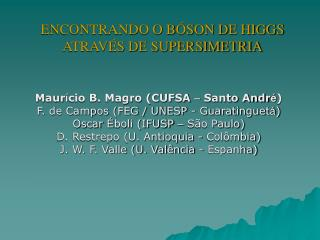Download PresentationENCONTRANDO O BÓSON DE HIGGS ATRAVÉS DE SUPERSIMETRIA

# ENCONTRANDO O BÓSON DE HIGGS ATRAVÉS DE SUPERSIMETRIA - PowerPoint PPT PresentationDownload Presentation## ENCONTRANDO O BÓSON DE HIGGS ATRAVÉS DE SUPERSIMETRIA

- - - - - - - - - - - - - - - - - - - - - - - - - - - E N D - - - - - - - - - - - - - - - - - - - - - - - - - - -
##### Presentation Transcript

1. ENCONTRANDO O BÓSON DE HIGGS ATRAVÉS DE SUPERSIMETRIA Maurício B. Magro (CUFSA – Santo André)F. de Campos (FEG / UNESP - Guaratinguetá)Oscar Éboli (IFUSP – São Paulo)D. Restrepo (U. Antioquia - Colômbia)J. W. F. Valle (U. Valência - Espanha)

2. SUPERSYMMETRY AND THE HIGGS BOSON • The CERN LHC will test the origin of mass and the nature of electroweak symmetry breaking  Higgs Boson  Hierarchy Problem • Supersymmetry (SUSY) is a possible solution to the hierarchy problem; • Weak scale Supersymmetry breaking unifies gauge coupling constants and can include gravity; • SUSY is broken via SuperGravity ↓↓↓↓ New physics beyond the Standard Model

3. THE BILINEAR R-PARITY MODEL IN mAMSB • In SUSY there is a multiplicative quantum number called R-parity: RP = (-1)2J+3B+L If R-parity is conserved: 1. SUSY particles are produced in pairs. 2. SUSY particles must decay into antother SUSY particles. 3. There is a stable SUSY particle: LSP (neutralino). • We introduced a bilinear term in the SUSY superpotential: εiLiHu that breaks R-parity explicitly.

4. Consequences Only one neutrino aquires mass at tree level. The neutrino mass spectrum can be cosistent with neutrino oscillation data, favouring  → . The neutrino mass is given by: with Λi = νi + εiνd The neutrino mixing angles are given by: and The lightest nuetralino is unstable, therefore can decay inside the present detector colliders  New signatures at colliders. The Neutralino branching ratio into Higgs is:

5. HIGGS SEARCH Neutralino Decays • It can decay through Higgs boson as: χ0→  H* .→ ν bb • The model backgrounds are: χ0→  Z* .→ ν bb and χ0.→ ν bb χ0→ τW.→ ν qq (mistaged b´s)

6. The LSP can live long enough to produce a displaced vertex at detectors. • The displaced vertices have litte if none background at LHC • We have considered a neutralino that lives long enough to decay out the primary vertex and before the tracking detectors. • We have applied the following kinematic cuts: 1. All charged tracks must be inside the region of |η| < 2.5; 2. We considered a b-tagging efficiency of either 50% or 30%; 3. We required an isolated electon(muon) with pt > 20(6) GeV; or a jet with pt > 100 GeV; or missing tranverse energy Etmiss > 100 GeV; 4. We required an invariant mass inside the range 100 GeV < MH < 125 GeV;

7. THE RESULTS • The parameters are: m1/2; m0; Tanβ = 10; sign() > 0; εi; Λi. - We have used SPheno code to calculate the R-Parity violating branching ratios and Pythia code to calculate the cross sections. - We required at least 5 background free events, consistent with neutrino data. Reach for LHC Reach for Tevatron 10 fb-1 (stars) and 100 fb-1 (squares) 2 fb-1 (stars) and 8 fb-1 (squares)

8. Results Reach for LHC Reach for Tevatron 10 fb-1 (stars) and 100 fb-1 (squares) 2 fb-1 (stars) and 8 fb-1 (squares) low efficienciy • For more details, see Phys. Rev. D80, 015002 (2009).